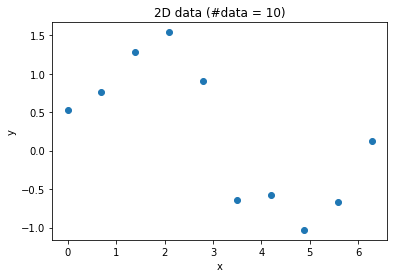HTML generated from Jupyter notebook: linear-regression-polygon-fitting-exercise.ipynb

# Exploring linear regression¶

In :
import numpy as np
import matplotlib.pyplot as plt
%matplotlib inline
import time
import pylab as pl
from IPython import display

np.random.seed(0)

In :
N = 10 # number of data points
x = np.linspace(0,2*np.pi,N)
y = np.sin(x) + np.random.normal(0,.3,x.shape)
plt.figure()
plt.plot(x,y,'o')
plt.xlabel('x')
plt.ylabel('y')
plt.title('2D data (#data = %d)' % N)
plt.show()## Complete the following tasks¶

1. Complete the following function
def polyfit(x,y,degree,delta):
"""
Fits a polynomial to 2D data (x,y)

Arguments:
x, y -- x and y data points
degree -- polynomial degree
delta -- regularization parameter

Returns:
Fitted parameters theta, where y = p(x) = \sum_{i=0}^degree \theta_i x^i
"""
1. Complete the following function
def polyeval(theta, x):
"""
Evaluates a 1D polynomial (i.e., fitted to 2D points (x,y))

Arguments:
x -- points at which we want to evaluate the polynomial
theta -- polynomial parameters

Returns:
p(x) -- where p(x) = \sum_{i=0}^degree \theta_i x^i
"""
1. Write down a routine that performs polygon fitting using gradient descent. Recall that the least squares cost is $J(\theta) = (\mathbf{X} \theta - \mathbf{Y})^T(\mathbf{X} \theta - \mathbf{Y})$.